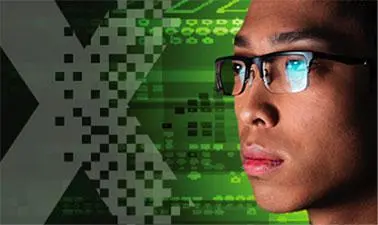There is 0 item now in your comparison listView a comparison list

# Computational Thinking and Big Data

Price
Free
21,870 people completed this program

## Overview

Computational thinking is an invaluable skill that can be used across every industry, as it allows you to formulate a problem and express a solution in such a way that a computer can effectively carry it out.

In this course, part of the Big Data MicroMasters program, you will learn how to apply computational thinking in data science. You will learn core computational thinking concepts including decomposition, pattern recognition, abstraction, and algorithmic thinking.

You will also learn about data representation and analysis and the processes of cleaning, presenting, and visualizing data. You will develop skills in data-driven problem design and algorithms for big data.

The course will also explain mathematical representations, probabilistic and statistical models, dimension reduction and Bayesian models.

You will use tools such as R and Java data processing libraries in associated language environments.

• Understand and apply advanced core computational thinking concepts to large-scale data sets
• Use industry-level tools for data preparation and visualisation, such as R and Java
• Apply methods for data preparation to large data sets
• Understand mathematical and statistical techniques for attracting information from large data sets and illuminating relationships between data sets

## Syllabus

Section 1: Data in R

Identify the components of RStudio; Identify the subjects and types of variables in R; Summarise and visualise univariate data, including histograms and box plots.

Section 2: Visualising relationships

Produce plots in ggplot2 in R to illustrate the relationship between pairs of variables; Understand which type of plot to use for different variables; Identify methods to deal with large datasets.

Section 3: Manipulating and joining data

Organise different data types, including strings, dates and times; Filter subjects in a data frame, select individual variables, group data by variables and calculate summary statistics; Join separate dataframes into a single dataframe; Learn how to implement these methods in mapReduce.

Section 4: Transforming data and dimension reduction

Transform data so that it is more appropriate for modelling; Use various methods to transform variables, including q-q plots and Box-Cox transformation, so that they are distributed normally Reduce the number of variables using PCA; Learn how to implement these techniques into modelling data with linear models.

Section 5: Summarising data

Estimate model parameters, both point and interval estimates; Differentiate between the statistical concepts or parameters and statistics; Use statistical summaries to infer population characteristics; Utilise strings; Learn about k-mers in genomics and their relationship to perfect hash functions as an example of text manipulation.

Section 6: Introduction to Java

Use complex data structures; Implement your own data structures to organise data; Explain the differences between classes and objects; Motivate object-orientation.

Section 7: Graphs

Encode directed and undirected graphs in different data structures, such as matrices and adjacency lists; Execute basic algorithms, such as depth-first search and breadth-first search.

Section 8: Probability

Determine the probability of events occurring when the probability distribution is discrete; How to approximate.

Section 9: Hashing

Apply hash functions on basic data structures in Java; Implement your own hash functions and execute, these as well as built-in ones; Differentiate good from bad hash functions based on the concept of collisions.

Section 10: Bringing it all together

Understand the context of big data in programming.

## Authored by